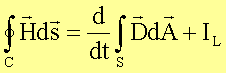# What is the equation of displacement current

The displacement current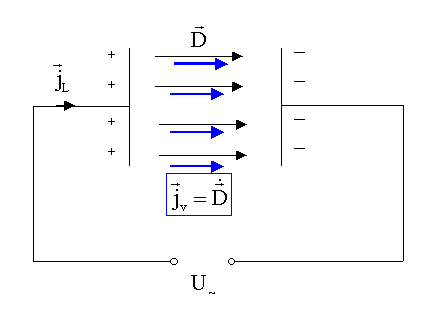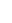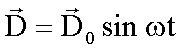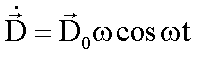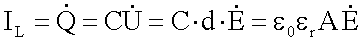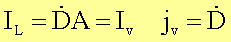The line current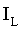settles in the plate capacitor as a displacement current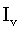away. The equality ofandapplies provided that the dielectric between the capacitor plates has no conductivity. Otherwise, a proportional line current is added to the displacement current. The current densities jv in the capacitor and jL. in the conductor are generally different, since the line cross-section is not the same as the cross-section A of the capacitor.

With the displacement current density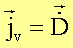the total current density expands at a certain cross-section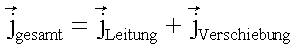This extends Maxwell's equation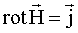toor in integral form (Oerstedt’s law)